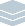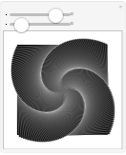Wolfram Archive
Wolfram Programming Lab is a legacy product.
All the same functionality and features, including access to Programming Lab Explorations, are available with Wolfram|One.
Start programming now. »# Spiraling Squares

Make an app for creating spiral designs by incrementally rotating squares in a stack.

Run the code to rotate a square by 30 degrees. Try different angles (e.g. 45 degrees):

This draws a default rectangle, which is a horizontal square:

Graphics[Rectangle[]]

This draws the square rotated:

Graphics[Rotate[Rectangle[], 30 Degree]]

Graphics[Rotate[Rectangle[], 30 Degree]]

Create an interface with a slider to rotate the square. Try angle ranges other than 360:

You can make anything interactive in the Wolfram Language with Manipulatefor example, this code:

Graphics[Rotate[Rectangle[], 30 Degree]]

First replace the numeric angle value with a variable named angle:

Graphics[Rotate[Rectangle[], angle Degree]]

Then wrap the expression with Manipulate and specify the range of the variable. Manipulate automatically constructs an interface with a slider that controls the value of the angle:

Manipulate[ Graphics[Rotate[Rectangle[], angle Degree]], {angle, 0, 360} ]

Manipulate[ Graphics[Rotate[Rectangle[], angle Degree]], {angle, 0, 360} ]

Make a stack of 10 squares rotated in 5-degree increments. Try other increments or other numbers of squares:

Table makes lists of elements, one for each value of a variable in a specified range. For example, this makes a list of even numbers:

Table[2 r, {r, 0, 10}]

This uses Table to make a stack of rectangles, each one rotated 5 degrees more than the one below it. It doesnt look like a stack of rectangles because you cant see their edges:

Graphics[{Table[Rotate[Rectangle[], r 5 Degree], {r, 0, 10}]}]

Make the edges white so you can see the individual rectangles in the stack:

Graphics[{EdgeForm[White], Table[Rotate[Rectangle[], r 5 Degree], {r, 0, 10}]}]

Graphics[{EdgeForm[White], Table[Rotate[Rectangle[], r 5 Degree], {r, 0, 10}]}]

Create an interface for changing the rotation increment between 0 and 45 degrees:

This draws a stack of rectangles:

Graphics[{EdgeForm[White], Table[Rotate[Rectangle[], r 5 Degree], {r, 0, 10}]}]

Make that expression interactive with Manipulate. Replace the numeric angle value with a variable named angle:

Graphics[{EdgeForm[White], Table[Rotate[Rectangle[], r angle Degree], {r, 0, 10}]}]

Then wrap the expression with Manipulate and specify the range of the angle variable:

Manipulate[ Graphics[{EdgeForm[White], Table[Rotate[Rectangle[], r angle Degree], {r, 0, 10}]}], {angle, 0, 360} ]

Manipulate[ Graphics[{EdgeForm[White], Table[Rotate[Rectangle[], angle r Degree], {r, 0, 10}]}], {angle, 0, 45} ]

Add another control for changing the number of squares:

The number of squares in this expression is determined by the value 10:

Manipulate[ Graphics[{EdgeForm[White], Table[Rotate[Rectangle[], angle r Degree], {r, 0, 10}]}], {angle, 0, 45} ]

Replace 10 by the variable n, and specify that n goes from 1 to 100 in steps of 1:

Manipulate[ Graphics[{EdgeForm[White], Table[Rotate[Rectangle[], angle r Degree], {r, 0, n}]}], {angle, 0, 45}, {n, 1, 100, 1} ]

Manipulate[ Graphics[{EdgeForm[White], Table[Rotate[Rectangle[], angle r Degree], {r, 0, n}]}], {angle, 0, 45}, {n, 1, 100, 1} ]

Make a sequence of progressively smaller squares. Try increments other than 5 degrees:

This draws a stack of squares rotated in 5-degree increments:

Graphics[{EdgeForm[White], Table[Rotate[Rectangle[], 5 r Degree], {r, 0, 10}]}]

This uses Scale to scale down the squares as they rotate. The scale factor is 1-r/10, which goes from 1 to 0 as r goes from 0 to 10. The effect is to scale the squares from full-sized down to nothing:

Graphics[{EdgeForm[White], Table[Scale[Rotate[Rectangle[], 5 r Degree], 1 - r/10], {r, 0, 10}]}]

Graphics[{EdgeForm[White], Table[Scale[Rotate[Rectangle[], 5 r Degree], 1 - r/10], {r, 0, 10}]}]

Put everything together. Find flower-like and other patterns:

Add scaling so that the rectangles get smaller as they rotate. Now you have a complete interface for exploring spiral designs:

Manipulate[ Graphics[{EdgeForm[White], Table[Scale[Rotate[Rectangle[], angle r Degree], 1 - r/n], {r, 0, n}]}], {angle, 0, 45}, {n, 1, 100} ]

Manipulate[ Graphics[{EdgeForm[White], Table[Scale[Rotate[Rectangle[], angle r Degree], 1 - r/n], {r, 0, n}]}], {angle, 0, 45}, {n, 1, 100} ]

Share Itmake an interactive website for exploring spiral designs:

Deploy the Manipulate to the Wolfram Cloud, where anyone with a browser can use it:

CloudDeploy[ Manipulate[ Graphics[{EdgeForm[White], Table[Scale[Rotate[Rectangle[], angle r Degree], 1 - r/n], {r, 0, n}]}], {angle, 0, 45}, {n, 1, 100} ], Permissions -> "Public" ]

Click the link in the output to visit the site.